# Asymptotes

An asymptote is a straight line that constantly approaches a given curve but does not meet at any infinite distance. In other words, Asymptote is a line that a curve approaches as it moves towards infinity.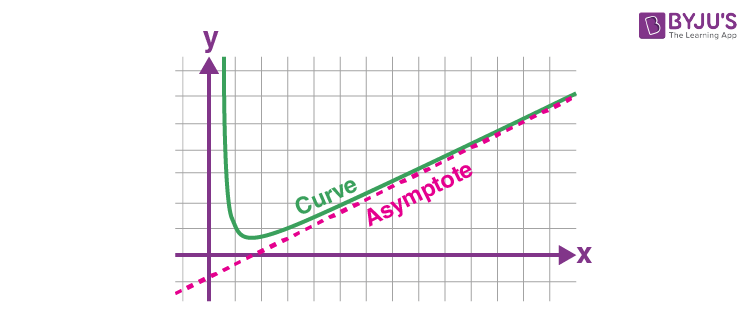The curves visit these asymptotes but never overtake them. The method opted to find the horizontal asymptote changes involves comparing the degrees of the polynomials in the numerator and denominator of the function. If both the polynomials have the same degree, divide the coefficients of the largest degree terms.

## Asymptotes Meaning

An asymptote of the curve y = f(x) or in the implicit form: f(x,y) = 0 is a straight line such that the distance between the curve and the straight line lends to zero when the points on the curve approach infinity.

There are three types of asymptotes namely:

• Vertical Asymptotes
• Horizontal Asymptotes
• Oblique Asymptotes

The point to note is that the distance between the curve and the asymptote tends to be zero as it moves to infinity or -infinity.

### Horizontal Asymptote

When x moves to infinity or -infinity, the curve approaches some constant value b, and is called a Horizontal Asymptote.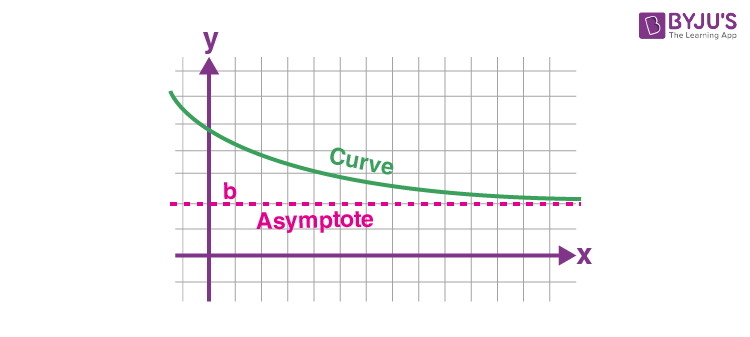### Vertical Asymptote

When x approaches some constant value c from left or right, the curve moves towards infinity (i.e.,∞)  , or -infinity (i.e., -∞) and this is called Vertical Asymptote.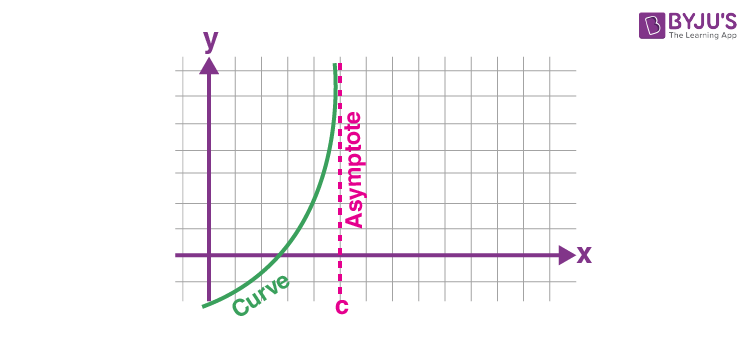### Oblique Asymptote

When x moves towards infinity (i.e.,∞)  , or -infinity (i.e., -∞), the curve moves towards a line y = mx + b, called Oblique Asymptote.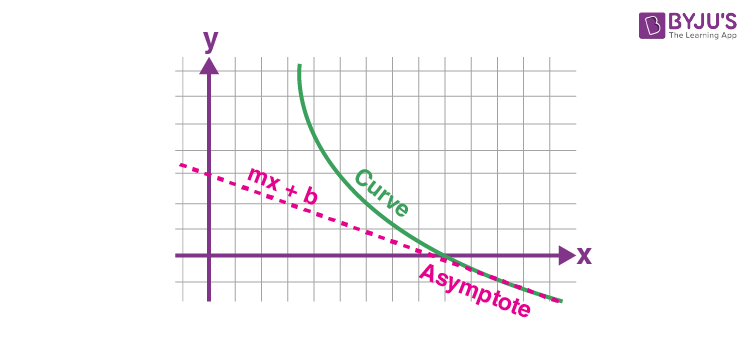Please note that m is not zero since that is a Horizontal Asymptote.

### Asymptote Equation

We know that the vertical asymptote has a straight line equation is x = a for the graph function y = f(x), if it satisfies at least one the following conditions:

$$\begin{array}{l}\lim_{x\rightarrow a-0}f(x)=\pm \infty\end{array}$$
or
$$\begin{array}{l}\lim_{x\rightarrow a+0}f(x)=\pm \infty\end{array}$$

Otherwise, at least one of the one-sided limit at point x=a must be equal to infinity.

For Oblique asymptote of the graph function y=f(x) for the straight-line equation is y=kx+b for the limit x → + ∞, if and only if the following two limits are finite.

$$\begin{array}{l}\lim_{x\rightarrow +\infty }\frac{f(x)}{x} = k\end{array}$$
and
$$\begin{array}{l}\lim_{x\rightarrow +\infty }[f(x)- kx] = b\end{array}$$

For horizontal asymptote, for the graph function y=f(x) where the straight line equation is y=b, which is the asymptote of a function x → + ∞, if the following limit is finite.

$$\begin{array}{l}\lim_{x\rightarrow +\infty }f(x) = b\end{array}$$

The above limit is same for x → – ∞,

Example:

Find the asymptotes of the function f(x) = (3x – 2)/(x + 1)

Solution:

Given,

f(x) = (3x – 2)/(x + 1)

Here, f(x) is not defined for x = -1.

Let us find the one-sided limits for the given function at x = -1.

$$\begin{array}{l}\lim_{x\rightarrow -a-0}f(x)=\lim_{x\rightarrow -1-0}\frac{3x-2}{x+1} =\frac{-5}{-0}=+\infty \\ \lim_{x\rightarrow -a+0}f(x)=\lim_{x\rightarrow -1+0}\frac{3x-2}{x+1} =\frac{-5}{0}=-\infty\end{array}$$

Therefore, the function f(x) has a vertical asymptote at x = -1.

Now, let us find the horizontal asymptotes by taking x → ±∞

$$\begin{array}{l}\lim_{x\rightarrow \pm\infty}f(x)=\lim_{x\rightarrow \pm\infty}\frac{3x-2}{x+1} = \lim_{x\rightarrow \pm\infty}\frac{3-\frac{2}{x}}{1+\frac{1}{x}} = \frac{3}{1}=3\end{array}$$

Therefore, the function f(x) has a horizontal asymptote at y = 3.

$$\begin{array}{l}k=\lim_{x\rightarrow +\infty}\frac{f(x)}{x}\\=\lim_{x\rightarrow +\infty}\frac{3x-2}{x(x+1)}\\ = \lim_{x\rightarrow +\infty}\frac{3x-2}{(x^2+x)}\\=\lim_{x\rightarrow +\infty}\frac{\frac{3}{x}-\frac{2}{x^2}}{1+\frac{1}{x}} \\= \frac{0}{1}\\=0\end{array}$$

Similarly, we can get the same value for x → -∞.

As k = 0, there are no oblique asymptotes for the given function.

### How to Find Horizontal Asymptotes?

To recall that an asymptote is a line that the graph of a function approaches but never touches. In the following example, a Rational function consists of asymptotes.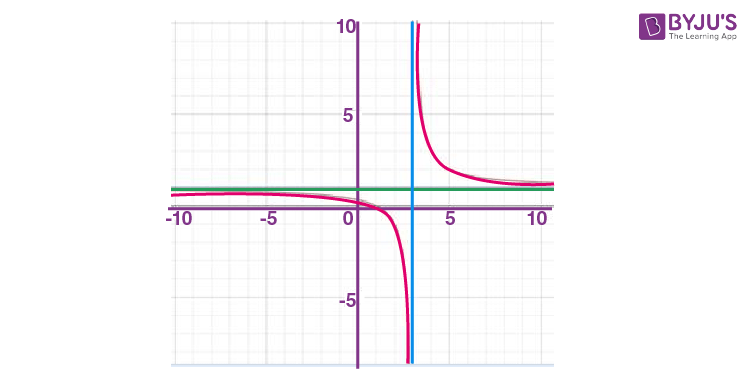In the above example, we have a vertical asymptote at x = 3 and a horizontal asymptote at y = 1. The curves approach these asymptotes but never visit them.

The method to identify the horizontal asymptote changes based on how the degrees of the polynomial in the function’s numerator and denominator are compared. Below are the points to remember to find the horizontal asymptotes:

• If the degree of the polynomials both in numerator and denominator is equal, then divide the coefficients of highest degree terms to get the horizontal asymptotes.
• If the degree of the numerator is less than the degree of the denominator, then the horizontal asymptotes will be y = 0.
• If the degree of the numerator is greater than the degree of the denominator, then there are no horizontal asymptotes.

### Asymptotes of a Hyperbola

Hyperbola contains two asymptotes. Two bisecting lines that are passing by the center of the hyperbola that doesn’t touch the curve are known as the Asymptotes. These can be observed in the below figure.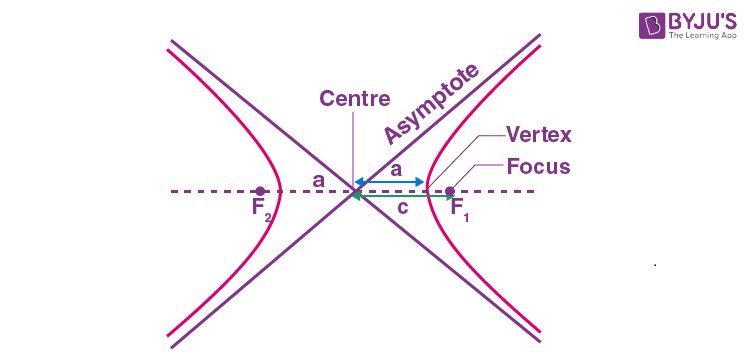If the centre of a hyperbola is (x0, y0), then the equation of asymptotes is given as: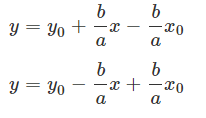If the centre of the hyperbola is located at the origin, then the pair of asymptotes is given as:

y = ±(b/a)x

That means,

y = (b/a)x

y = -(b/a)x

Let us see some examples to find horizontal asymptotes.

### Asymptote Examples

Example 1:

Find the horizontal asymptotes for f(x) = x+1/2x

Solution:

Given, f(x) = (x+1)/2x

Since the highest degree here in both numerator and denominator is 1, therefore, we will consider here the coefficient of x.

Hence, horizontal asymptote is located at y = 1/2

Example 2:

Find the horizontal asymptotes for f(x) = x/x2+3

Solution:

Given, f(x) = x/x2+3

Since we can see here the degree of the numerator is less than the denominator, therefore, the horizontal asymptote is located at y = 0.

Example 3:

Find the horizontal asymptotes for f(x) =(x2+3)/x+1

Solution:

Given, f(x) =(x2+3)/x+1

As you can see, the degree of the numerator is greater than that of the denominator. Hence, there is no horizontal asymptote.